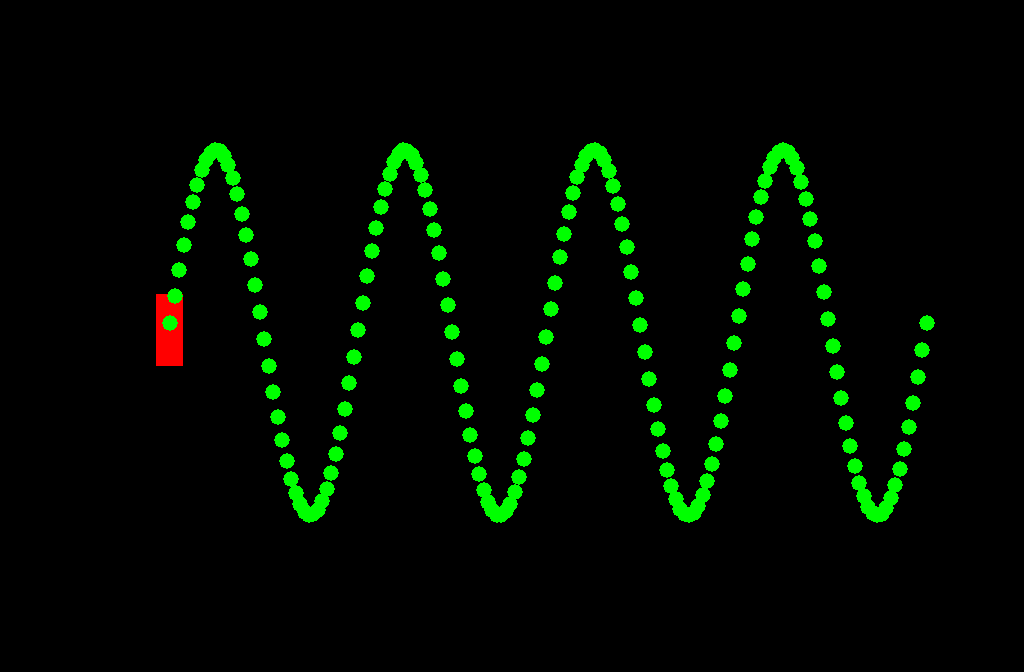Animations of Acoustic Waves

1.

WAVE BASICS

2.

WAVE INTERACTION

3.

STANDING WAVES

4.

HEARING SOUNDS

5.

FURTHER CONCEPTS

6.

LEARN MOREUNIVERSITY OF SOUTHAMPTONWAVE BASICSSummary

THE NATURE OF WAVES• A wave is a disturbance in a medium.

• It carries energy from one point to another without transferring matter.

• There are two main types: Longitudinal and Transverse.

• Sound waves are an example of longitudinal waves.

• Typically, sound waves travel at about 340 meters per second in air at room temperature and pressure.

WAYS OF SHOWING WAVES

We use three ways of representing the propagation of waves:

• Dots.

• Colour maps.

• Line graphs.

WAVELENGTH-FREQUENCY RELATION

Frequency, f, wavelength, λ, and wave velocity, v, are mathematically related by:

POINT SOURCES, INVERSE SQUARE LAW

The energy carried by waves that radiate uniformly in all directions from a point source decay as the inverse of the square of the distance from the point source. This is known as the inverse square law.WAVE BASICS